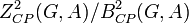# Cyclicity-preserving subgroup of second cohomology group for trivial group action

Jump to: navigation, search

## Definition

Suppose$G$ is a group and$A$ is an abelian group. The group that we call the cyclicity-preserving subgroup of second cohomology group for trivial group action is defined in the following equivalent ways:

1. It is the subgroup of the second cohomology group for the trivial action of$G$ on$A$, comprising those cohomology classes that can be represented by a cyclicity-preserving 2-cocycle for trivial group action.
2. It is the quotient group of the group of cyclicity-preserving 2-cocycles for trivial group action by the group of cyclicity-preserving 2-coboundaries for trivial group action.

In symbols, this group is denoted$H^2_{CP}(G,A)$ and is defined as$Z^2_{CP}(G,A)/B^2_{CP}(G,A)$.